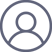余高奇2022-9-29 17:37

本文拟解析理想气体恒温膨胀过程中的可逆过程与不可逆过程的异同，供参考.

例：某双原子理想气体1mol从始态350K, 200kPa经过如下两个不同过程均达到终态压强为50kPa，计算各自过程的ΔU、ΔH、ΔSW（体积功）及Q.

(1)恒温可逆膨胀；

(2)恒温反抗50kPa恒外压不可逆膨胀.

依题：始态 T1=350K, p1=200kPa,

V1=nRT1/p1=1mol×8.314J·mol-1·K-1×350K/(200kPa)=14.550dm3.

终态T2=350K, p2=50kPa,

V2=nRT2/p2=1mol×8.314J·mol-1·K-1×350K/(50kPa)=58.198dm3.

另：因理想气体内能与焓仅为温度函数，理想气体pVT变化的恒温过程，ΔUH=0.

1. 恒温可逆膨胀

对于可逆过程，体积功：

W1=-∫p·dV=-∫（nRT/V)·dV

=nRT·ln(V1/V2)

=1mol×8.314J·mol-1·K-1×350K×ln(14.550dm3/58.198dm3)

=-4.034kJ

依热力学第一定律可得：ΔU= W1+Q1       （1）

由式（1）可得：Q1=-W1=4.034kJ

另对于恒温可逆过程，ΔS1=Q1/T=4.034kJ/350K=11.53J·K-1

2. 恒温不可逆膨胀

对于不可逆过程，体积功：

W2=-∫pe·dV=-pe·(V2-V1)

=-50kPa×(58.198dm3-14.550dm3)

=-2.182kJ

由式（1）可得：Q2=-W2=2.182kJ

由于（1）恒温可逆膨胀与（2）恒温不可逆膨胀，系统的始、末态均相同，两过程的熵变相同，即：

ΔS2S1=11.53J·K-1

3. 热力学计算结果对比

(1)与（2）热力学结果对比参见如下表1.

表1. 理想气体恒温可逆与不可逆膨胀过程的热力学性质对比

 热力学过程 ΔU(/J) ΔH(/J) ΔS(/J·K-1) W(/J) Q(/J) 恒温可逆膨胀 0 0 11.53 -4.034 4.034 恒温不可逆膨胀 0 0 11.53 -2.182 2.182

表1结果显示，可逆过程与不可逆过程的体积功与热量不相同.

3.1 平衡态热力学的解释

平衡态热力学认为系统类似一个黑箱子，其热力学能的结构不可能知道；

此时只能通过环境所获取的体积功（δW=-pe·dV）及热量（δQ），间接确定系统的内能改变量, 即：dU= -pe·dV+δQ .

体积功的计算通常包括如下三种情况：

①自由膨胀，δW=-pe·dV=0；

②恒外压膨胀，W=-∫(pe·dV)=-pe·（V2-V1）=-pe·ΔV

③恒温可逆膨胀，W=-∫(pe·dV)

=-∫(p·dV)=-∫（nRT/V)·dV=nRT·ln(V1/V2)

3.2 平衡态热力学解释存在的问题

存在问题主要包括以下两点：

首先为什么真实热力学过程的熵变只能通过可逆过程（虚拟过程）来计算？

其次为什么可逆过程的体积功需用δW=-p·dV计算？不可逆过程，“-p·dV”不存在了吗？意义是什么？

天津大学物理化学教研室. 物理化学（上册，第四版）.北京：高等教育出版社,2001,12:97